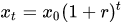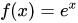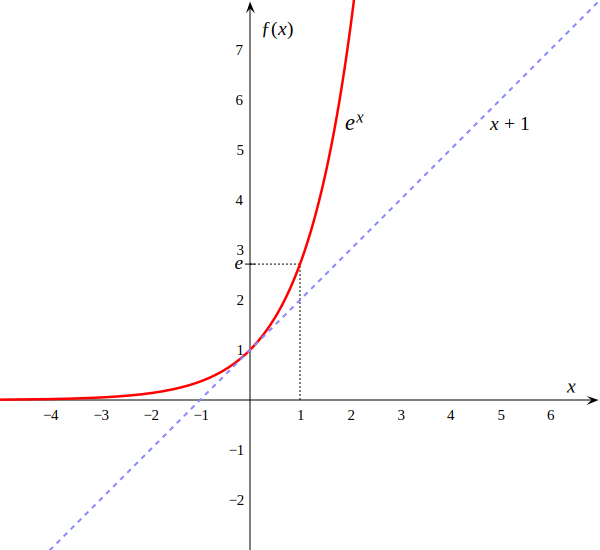# Exponential growth/decay formula calculator online free

Exponential growth/decay formula calculator online free

Exponential growth/decay formula

x(t) = x0 × (1 + r) t

x(t) is the value at time t.

x0 is the initial value at time t=0.

r is the growth rate when r > 0 or decay rate when r < 0, in percent.

t is the time in discrete intervals and selected time units.

## Exponential growth calculator

Enter the initial value x0, growth rate r and time interval t and press the Calculate button:

%

## Example

x0 = 50

r = 4% = 0.04

t = 90 hours

x(t) = x0 × (1 + r) t = 50×(1+0.04)90 = 1706

## Definition

Exponential growth is a process that increases quantity over time. It occurs when the instantaneous rate of change (that is, the derivative) of a quantity with respect to time is proportional to the quantity itself. Described as a function, a quantity undergoing exponential growth is an exponential function of time, that is, the variable representing time is the exponent (in contrast to other types of growth, such as quadratic growth).
The formula for exponential growth of a variable x at the growth rate r, as time t goes on in discrete intervals (that is, at integer times 0, 1, 2, 3, ...), iswhere x0 is the value of x at time 0. The same expression is also taken into consideration by the best exponential growth calculator to calculate the most authentic results.

The growth of a bacterial colony is often used to illustrate it. One bacterium splits itself into two, each of which splits itself resulting in four, then eight, 16, 32, and so on. The rate of increase keeps increasing because it is proportional to the ever-increasing number of bacteria. Growth like this is observed in real-life activity or phenomena, such as the spread of virus infection, the growth of debt due to compound interest, and the spread of viral videos. In real cases, initial exponential growth often does not last forever, instead slowing down eventually due to upper limits caused by external factors and turning into logistic growth.

## Example graph

Graph of the exponential function(red) with the tangent (light blue dotted line) through the point 0/1Source: Wikipedia

Exponent calculator »

Currently, we have around 5661 calculators, conversion tables and usefull online tools and software features for students, teaching and teachers, designers and simply for everyone.